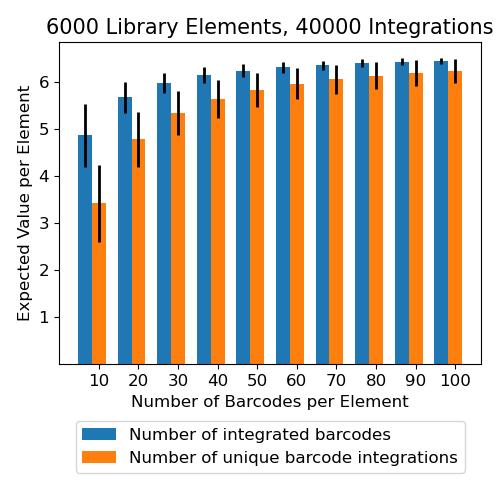# Unique Barcode Integration Calculator

This is a tool to help investigators design massively parallel genome-integrated assays. The tool assumes an experimental design in which a library of DNA elements is barcoded and then integrated into the genomes of cells, where the functions of all elements are then assayed in parallel. The libraries are constructed such that each DNA element is present multiple times in the library, each time attached to a different unique barcode. Having multiple barcodes per element provides redundant measurements of each DNA element in the library.

In these experiments, three critical variables to consider are the number of DNA elements in the library $$E$$, the number of barcodes per element $$B$$, and the number of genome integrations $$I$$. The values of $$E$$, $$B$$, and $$I$$ determine how many barcodes an investigator can expect to recover in the experiment. Because the ability to detect and remove outliers is maximized when each barcode represents a single genome integration, we recommend trying to maximize the fraction of recovered barcodes that represent unique integrations, not just the total number of recovered barcodes.

Given input values for $$E$$, $$B$$, and $$I$$, this tool will compute (1) the expected number of barcodes recovered per element in the library and (2) the number of barcodes that represent unique integrations. The calculations assume that each integration is a random Poisson event with a mean specified by the total number of integrations divided by the total number of barcodes, i.e. $${I \over EB}$$. The tool allows users to specify values for each input variable. Users may also specify a range of values for one of the input variables.

## Inputs:

The radio button allows you to enter a minimum and maximum value for one parameter. Ten values will be evenly sampled across the provided range.

-
-
-

## Results:

In an ideal experiment, the number of unique barcode integrations is the same as the number of integrated barcodes.

Number of Elements in Library Number of Barcodes per Element Number of Integrations Total Number of Barcodes in Library Mean Number of Integrations per Barcode Number of Integrated Barcodes per Element Number of Unique Barcode Integrations per Element
6000 10 40000 60000 0.67 4.87 3.42
6000 20 40000 120000 0.33 5.67 4.78
6000 30 40000 180000 0.22 5.98 5.34
6000 40 40000 240000 0.17 6.14 5.64
6000 50 40000 300000 0.13 6.24 5.83
6000 60 40000 360000 0.11 6.31 5.97
6000 70 40000 420000 0.10 6.36 6.06
6000 80 40000 480000 0.08 6.40 6.13
6000 90 40000 540000 0.07 6.43 6.19
6000 100 40000 600000 0.07 6.45 6.24Please report all bugs to Ryan Friedman at ryan.friedman@wustl.edu